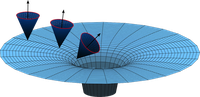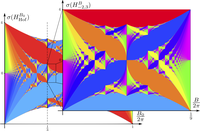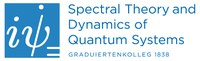Department of Mathematics

# People and Institutions

The master program in Mathematical Physics is jointly organized by the Mathematics Department and the Physics Department in Tübingen. To give you an impression of the team behind this program, here is a list of the people involved and a brief sketch of their research interests.

In the Mathematics Department, there are two groups whose members have research interests directly in the field of Mathematical Physics. They will be responsible for the core courses in Mathematical Physics.

## "Mathematical Relativity" groupProf. Carla Cederbaum -- Mathematical relativity, geometric analysis, differential geometry

Prof. Gerhard Huisken -- Geometric evolution equations, mathematical relativity, multilinear structures in differential geometry

Prof. Frank Loose -- Differential geometry, mathematical relativity

## "Mathematical quantum theory" groupJProf. Angela Capel Cuevas -- Quantum information theory, non-commutative functional inequalities and open quantum many-body systems

Dr. Stefan Keppeler -- Semiclassical analysis, diagrammatic group representations

Prof. Dr. Marius Lemm -- Many-body quantum theory, Schrödinger operators, random matrices

Prof. Peter Pickl -- Effective descriptions of many-body systems, propagation of chaos, mathematical statistical physics

Prof. Stefan Teufel -- Asymptotic and semiclassical analysis, geometry and topology in quantum systems, dimensional reduction

Prof. Roderich Tumulka -- Quantum statistical physics, foundations of quantum theory

In addition, the following members of the Mathematics Department and the Physics Department will also contribute to the course program and to the supervision of theses:

# Further InstitutionsThe research training school Spectral theory and dynamics of quantum systems funded by the German Science Foundation (DFG) is a joint PhD program with the University of Stuttgart specifically geared towards mathematical quantum physics. The scientific program of this school will be open also to the master students in Mathematical Physics.